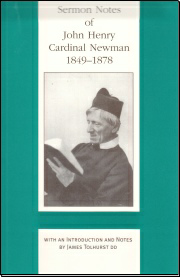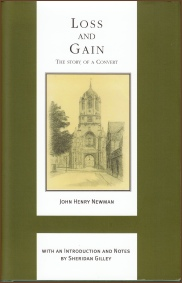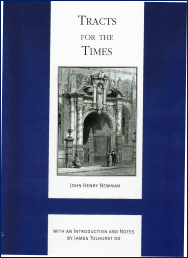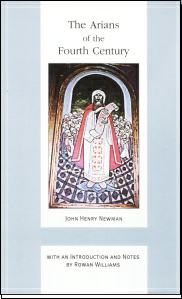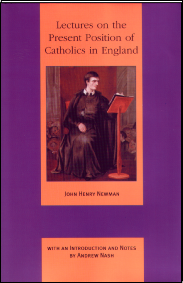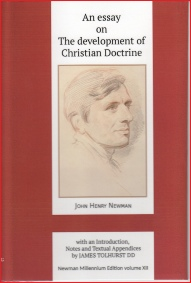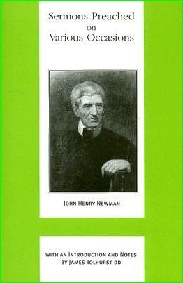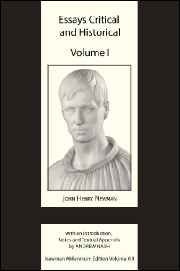﻿ NewmanBlessed John Henry Newman

The Works of Blessed John Henry Newman

The Birmingham Oratory Millennium Edition

Series Editor: James Tolhurst DD

An admirable new edition—The Catholic Herald

This series is warmly welcomed—New Directions

 Vol XII AN ESSAY ON THE DEVELOPMENT OF CHRISTIAN DOCTRINEIntroduced and edited by James Tolhurst Newman’s Essay on the Development of Christian Doctrine is one of the most ground-breaking and influential theological works since the Reformation. Written in the lead up to Newman’s conversion from the Anglican to the Catholic Church, it traces how early Christianity developed into Catholicism and has been described as doing for theology what Darwin did for biology. It is frequently referenced in debates about how Church teaching develops—what is authentic development and what isn’t. “A more intimate apprehension of original dogma” was John Henry Newman’s verdict on his Essay on the Development of Christian Dogma. He completed it in 1845 and thirty-three years later thoroughly revised it. The two versions are here compared in a new edition, tracing the process of Newman’s developing thoughts—with footnotes and appendices to bring out the importance of this seminal work, which in theological terms ranks alongside The Origin of Species. This is the first critical edition of the Essay. The editor, Newman scholar James Tolhurst, provides detailed notes on the many patristic and historical references in the text which may baffle the modern reader. In an illuminating Introduction he sets the historical context and summarises Newman’s idea of development. He also teases out the many revisions which Newman made to his original 1845 text when he revised it in 1878. The result is an edition which makes this classic text accessible both for Newman scholars and for the general reader. 978 178182 012 4  £35hb Other volumes in this series Vol I LECTURES ON THE PRESENT POSITION OF CATHOLICS Introduced and edited by Andrew Nash 978 085244 443 6   £25hb Vol II SERMONS NOTES Introduced and edited by James Tolhurst 978 085244 444 3   £25hb Vol III RISE AND PROGRESS OF THE UNIVERSITIES AND BENEDICTINE ESSAYS Introduced and edited by Katherine Tillman 978 085244 449 8   £25hb Vol IV ARIANS OF THE FOURTH CENTURY Introduced and edited by Archbishop Rowan Williams 978 085244 448 1   £25hb Vol V CHURCH OF THE FATHERS Introduced and edited by Frank McGrath 978 085244 447 4   £25hb Vol VI DISCOURSES ADDRESSED TO MIXED CONGREGATIONS Introduced and edited by James Tolhurst 978 085244 455 9   £25hb Vol VII DISCUSSIONS AND ARGUMENTS Introduced and edited by Gerard Tracey 978 085244 453 5   £25hb Vol VIII TWO ESSAYS ON BIBLICAL AND ON ECCLESIASTICAL MIRACLES Introduced by Bishop Geoffrey Rowell Edited by James Tolhurst 978 085244 456 6   £25hb Vol  IX SERMONS PREACHED ON VARIOUS OCCASIONS Introduced and edited by James Tolhurst 978 085244 408 5   £25hb Vol X TRACTS FOR THE TIMES Introduced and edited by James Tolhurst 978 085244 749 9  £25hb Vol XI LOSS AND GAINEdited and Introduced by Sheridan Gilley 978 085244 410 8  £25hb
View Basket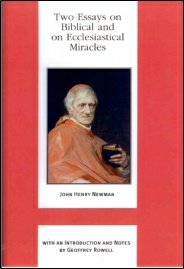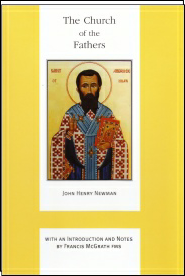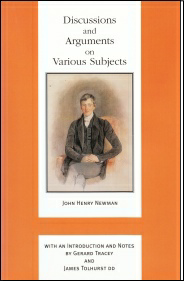S E R M O N S P R E A C H E D O N V A R I O U S O C C A S I O N S ( V o l. I X )

J o h n H e n r y N e w m a n

I n tr o d u c ti o n a n d N o t e s b y J a m e s T o l h u r st D D

J o h n H e n r y N e w m a n p o i n ts o u t i n t h e P r e f a c e t o t h e o ri g i n a l e d it i o n , t h a t h is s e ri e s o f S e r m o n s P r e a c h e d o n V a ri o u s O c c a si o n s w a s 't h e r e s u lt o f e x t e r n a l ci r c u m st a n c e s r a t h e r t h a n o f a n y s e t p u r p o s e o f h is o w n '. T h e p a rt ic u l a r e x t e r n a l ci r c u m st a n c e s w e r e t h e e st a b li s h m e n t o f t h e C a t h o li c U n i v e r si t y o f Ir el a n d ( w h ic h a c c o u n t e d f o r t h e fi r st ei g h t s e r m o n s) , t h e r e st o r a ti o n o f t h e C a t h o li c h ie r a r c h y i n E n g l a n d a n d W a le s (s e r m o n s 9 a n d 1 0 ), t h e R is o r g i m e n t o i n It a ly a n d it s r e p e r c u s si o n s o n t h e p a p a c y (s e r m o n 1 5 ) a n d t h e d e a t h o f t w o fr ie n d s, D r W e e d a ll a n d J a m e s H o p e S c o tt (s e r m o n s 1 3 a n d 1 4 ). N e w m a n a d d e d h is t w o -p a rt s e r m o n o n S t P h ill i p (s e r m o n 1 2 ) t o m a k e u p t h e t o t a l i n t h e fi r st e d it i o n .

T h e s e r m o n s o n t h e si t u a ti o n o f t h e C h u r c h i n E n g l a n d a n d W a le s, a n d t h e n o f t h e p a p a c y it s el f i n It a ly , r e fl e c t t h e t u m u lt u o u s ti m e s o f t h e m i d -n i n e t e e n t h c e n t u r y : a d e fi n i n g o f t h e r o le o f C a t h o li ci s m i n t h e d e v el o p m e n t o f t h e m o d e r n w o rl d .

9 7 8 0 8 5 2 4 4 4 0 8 5 4 0 8 p a g e s £ 2 5 h b